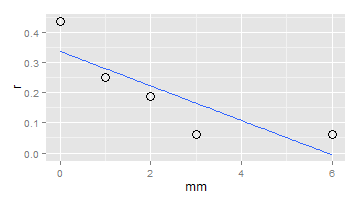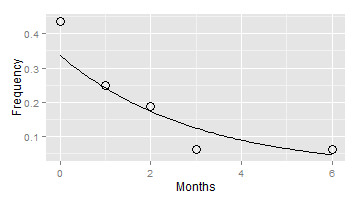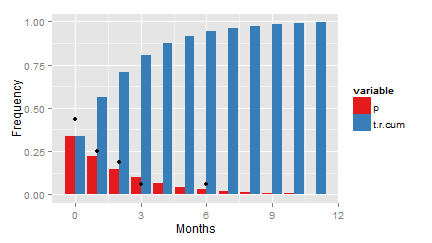# Predicting human resources in small business

It may leave you only cautiously optimistic knowing when it’s likely to interview the right candidate for recruitment, but it is better than nothing. One common issue businesses, and to a greater extent small businesses, face in managing their HR policies is staff turnover. With a local job market - in full swing in our sector and city contrary to common and current trends in Italy – that ensures employees quick and viable employment alternatives, than most other sectors, and especially in the case of highly skilled jobs where training costs can be rather high, businesses are left with a hefty penalty when an employee resigns.

Considering that this circumstance is somewhat raising staff bargaining power, the ‘wages’ item in a business’ cost and income account gets negatively impacted. Two considerations can be done about HR implications for reviewing employment policies. One is that there are exogenous given variables that can’t be changed and endogenous variables that can be tweaked at the manager’s heart content and the other is that decisions are always under uncertain conditions and that outcomes are only likely.

After 16 recruiting campaigns completed in the last 5 years – during which whenever a vacancy was to be filled a post was advertised on-line – our staff scoreboard looks like the following table:

Month Frequency
0 7/16
1 4/16
2 3/16
3 1/16
6 1/16

In 7 out of 16 campaigns we found the right candidate within the first month after posting the job vacancy, in 4 out of 16 withing the second month, etc. The table above shows the delay in finding the right candidate and the frequency of occurrence: zero means there was no delay in number of months between posting on line and interviewing the suitable candidate, as 1 means there was one month of delay in picking the right one.

At breakfast time on a dreary Sunday morning while sipping a cup of Earl Grey tea, I started a session on now my customary daily companion RStudio and punched in the following lines setting off for an unorthodox exercise in probability estimation:

````mm <- c(0, 1, 2, 3, 6)`
```r <- c(7/16, 4/16, 3/16, 1/16, 1/16)
cbind(mm, r)
``````
``````##      mm      r
## [1,]  0 0.4375
## [2,]  1 0.2500
## [3,]  2 0.1875
## [4,]  3 0.0625
## [5,]  6 0.0625
``````

Seeing them in column format didn’t add much and it was clearly necessary to plot the data:

``````library(ggplot2)
pl <- ggplot(data.frame(mm, r), aes(x = mm, y = r))
pl + geom_point(size = I(4), shape = 1) + stat_smooth(method = "lm", se = FALSE)
``````The plot above shows a clear decreasing pattern that made me think to fit the data with a regression, something whispering in my ear to use the log.

``````fit <- lm(log(r) ~ mm)
summary(fit)
``````
``````##
## Call:
## lm(formula = log(r) ~ mm)
##
## Residuals:
##       1       2       3       4       5
##  0.2616  0.0345  0.0794 -0.6866  0.3111
##
## Coefficients:
##             Estimate Std. Error t value Pr(>|t|)
## (Intercept)   -1.088      0.318   -3.42    0.042 *
## mm            -0.333      0.101   -3.30    0.046 *
## ---
## Signif. codes:  0 '***' 0.001 '**' 0.01 '*' 0.05 '.' 0.1 ' ' 1
##
## Residual standard error: 0.463 on 3 degrees of freedom
## Multiple R-squared:  0.784,  Adjusted R-squared:  0.713
## F-statistic: 10.9 on 1 and 3 DF,  p-value: 0.0456
``````

The regression yields significant coefficients that allow us to rule out coincidence. A further plotting effort to draw the curve just fitted I noticed revealed an apparently familiar frequency distribution:

``````fit_fun <- function(x) {
exp(fit\$coef) * exp(fit\$coef * x)
}
pl + geom_point(size = I(4), shape = 1) + stat_function(fun = fit_fun) + xlab("Months") +
ylab("Frequency")
``````Which looks like a negative binomial distribution! Too good to be true, I recall from a recent econometric review that the likelihood of a success in each given trial is the same and equals the intercept of my fitted regression curve:

``````fit\$coef
``````
``````## (Intercept)
##       -1.09
``````

Thus the probability of finding the right candidate in each given month, according to the binomial negative distribution should be 0.337.

``````r.prob <- exp(fit\$coef)
r.prob
``````
``````##       0.337
``````

If the probability of interviewing the right candidate is 0.337 each month then we can easily compute the probability of finding her or him only during the second month. It’s clearly the probability of a failure during the first month times the probability of a success in the second month:

``(1 - 0.337) * 0.337 ``
``## 0.223 ``

0.223 which is a smaller than 0.337. Similarly, the probability of seeing a success (i.e. interviewing the right candidate) only during the third month is 0.148. What we notice is that as time goes by into the recruiting campaign the likelihood of finding the right candidate diminishes only because the probability of having already found her or him in the previous months rises. Let’s compute the probability and the cumulated probability according to the binomial negative distribution over 12 months and display them in column format:

``````MONTH <- seq(0, 11, by = 1)
t.r <- dnbinom(MONTH, size = 1, prob = r.prob)
t.r.cum <- cumsum(t.r)
options(digits = 3)
cbind(t.r, t.r.cum)
``````
``````##           t.r t.r.cum
##  [1,] 0.33680   0.337
##  [2,] 0.22337   0.560
##  [3,] 0.14814   0.708
##  [4,] 0.09824   0.807
##  [5,] 0.06516   0.872
##  [6,] 0.04321   0.915
##  [7,] 0.02866   0.944
##  [8,] 0.01901   0.963
##  [9,] 0.01260   0.975
## [10,] 0.00836   0.984
## [11,] 0.00554   0.989
## [12,] 0.00368   0.993
``````

Building a data frame with the computed data about the density and cumulated frequencies, melting and displaying it is useful if you want to plot the data using ggplot2 package:

``````df <- data.frame(m = MONTH, p = t.r, t.r.cum)
library(reshape2)
df.m <- melt(df, id = c("m"))
``````
``````##   m variable  value
## 1 0        p 0.3368
## 2 1        p 0.2234
## 3 2        p 0.1481
## 4 3        p 0.0982
## 5 4        p 0.0652
## 6 5        p 0.0432
``````

Let’s build another data frame (df.1) with the actual data from the recruiting campaigns:

``````df.1 <- data.frame(mm = mm, r)
``````
``````##   mm      r
## 1  0 0.4375
## 2  1 0.2500
## 3  2 0.1875
## 4  3 0.0625
## 5  6 0.0625
``````

And at last we are ready for plotting it all in using ggplot2:

``````rr.pl <- ggplot()
rr.pl + geom_bar(data = df.m, aes(m, value, fill = variable), stat = "identity",
position = "dodge") + scale_fill_brewer(palette = "Set1") + geom_point(data = df.1,
aes(mm, r)) + xlab("Months") + ylab("Frequency")
``````The mean value of a negative distribution is (1-P)/P which in the present case is:

``````(1 - r.prob)/r.prob
``````
``````##  1.97
``````

Thus, we can reasonably expect to find the suitable candidate at the third month (1.97 + 1.00), since the first month counts as 0.

The whole HR compensation package could be critically reviewed since whenever an employee resigns the company suffers a cost in terms of unrealized revenues until it finds a new employee. This simple, though meaningful, analysis shows that two lines of intervention can be recommended: one is to activate the necessary mechanisms to improve the set of incentives to retain employees and the other is to optimize the recruiting campaign to shorten the time needed to find the right candidate.

Posted in Economics, Management, R-stats

### TweetsRodolfo Vanzini
@RodolfoVanzini